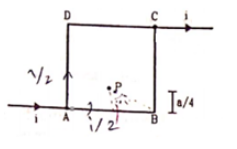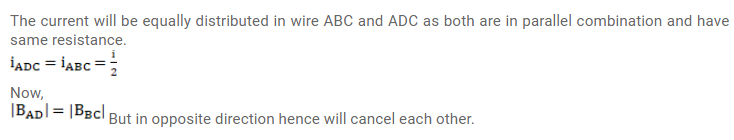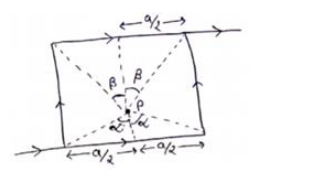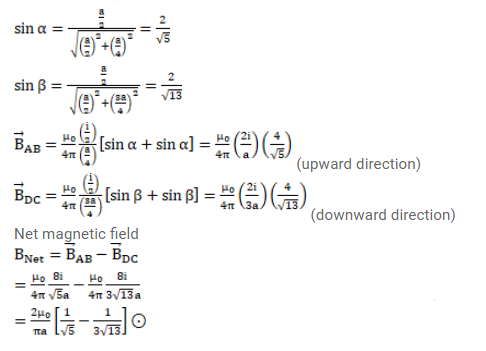# Figure shows a square loop of edge a made of a uniform wire.

Question:

Figure shows a square loop of edge a made of a uniform wire. A current $i$ enters the loop at the point $A$ and leaves it at the point C. Find the magnetic field at the point $P$ which is on the perpendicular bisector of $A B$ at a distance a/ 4 from it.Solution: